rus
Лас-Пальмас-де-Гран-...
03:27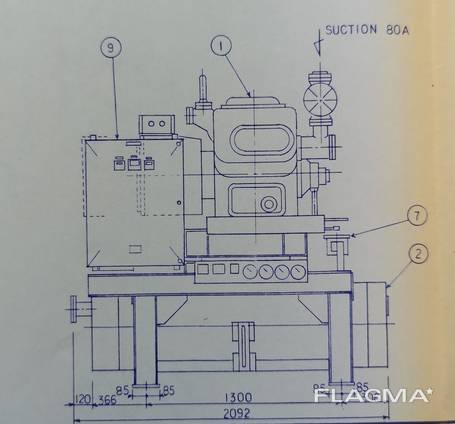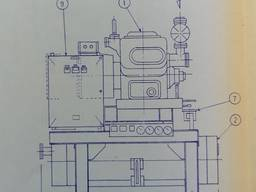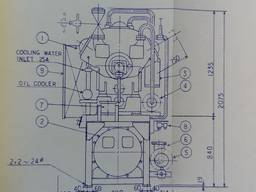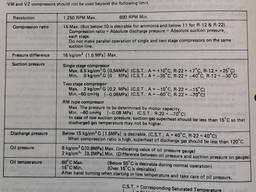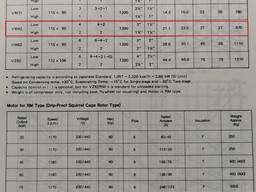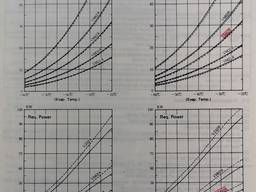Добавлено: 5 августа 2019, 17:46

# Hasegawa VM42 RM Compound Compressor and Spare Parts

Цена по запросу
Ship Chandler
director
Arnoma nesw, SL,  Лас-Пальмас-де-Гран-Канария, ES
на Флагма с 2 августа 2019

## Описание

Hasegawa VM42 RM Compound Compressor and Spare Parts

Model VM42
Cylinders: 4+2
RPM (Max): 1200
Bore In.: 115mm
Stroke In. 90mm
Unloading Steps 4-2
Suction In. 80mm
Discharge Low Stage In.: 40 mm
Suction High Stage In.: 40 mm
Discharge In.: 32 mm
Net Weight kg 1290
Oil Charge Litres: 25

VM42RM compressors should not be operated beyond the following limits:

Compressor RPM: Max. = 1250 rpm (VM =1500rpm) Min. = 600 rpm
Compression ratio* Max. = 15 Target Max: R717 – 10, R22 – 11
Pressure difference Max. = 227 psi (16.47 kg/cm″) [1.6MPa]

Suction pressure, Two stage compressors:
Max. 28 psig (C. S. T: R717 = 14°F, R22 = 5°F)
Max. 0.2 MPaG (C. S. T: R717 = -10°C, R22 = -15°C)
Min. 11 psig (C. S. T: R717 = -76°F, R22 = -94°F)
Min. -0.08MPaG (C. S. T: R717 = -60°C, R22 = -70°C)
RM type compressor
Max. Determined by motor capacity.
Min. 11 psig (C. S. T: R22 = -94°F)
Min. -0.08MPaG (C. S. T: R22 = -70°C)

Discharge pressure Below:
213 psig (C. S. T: R717 = 104°F, R22 = 104°F)
1.5 MPaG (C. S. T: R717 = 40°C, R22 = 40°C)

When compression ratio is high, temperature of discharge gas should be less than 248°F.

Oil Pressure:
Max. = 114 psig Min. = 28 psi Differential
Max. = 8kg/cm2G Min. = 2kg/cm2 Differential

Oil Temperature:
Max. = 140°F Min. = 59°F
Max. = 60°C Min. = 15°C
Normal operation = 131°F Normal operation = 55°C

Номер объявления: 1769771   |  просмотров: 8
Arnoma nesw, SL,  Лас-Пальмас-де-Гран-Канария, ES
на Флагма с 2 августа 2019
Свяжитесь с компанией
Ship Chandler
director
По телефонам
В мессенджерах
Скажите, что нашли объявление на Флагма
Написать сообщение
Выбрать раздел
×
Вход в личный кабинет